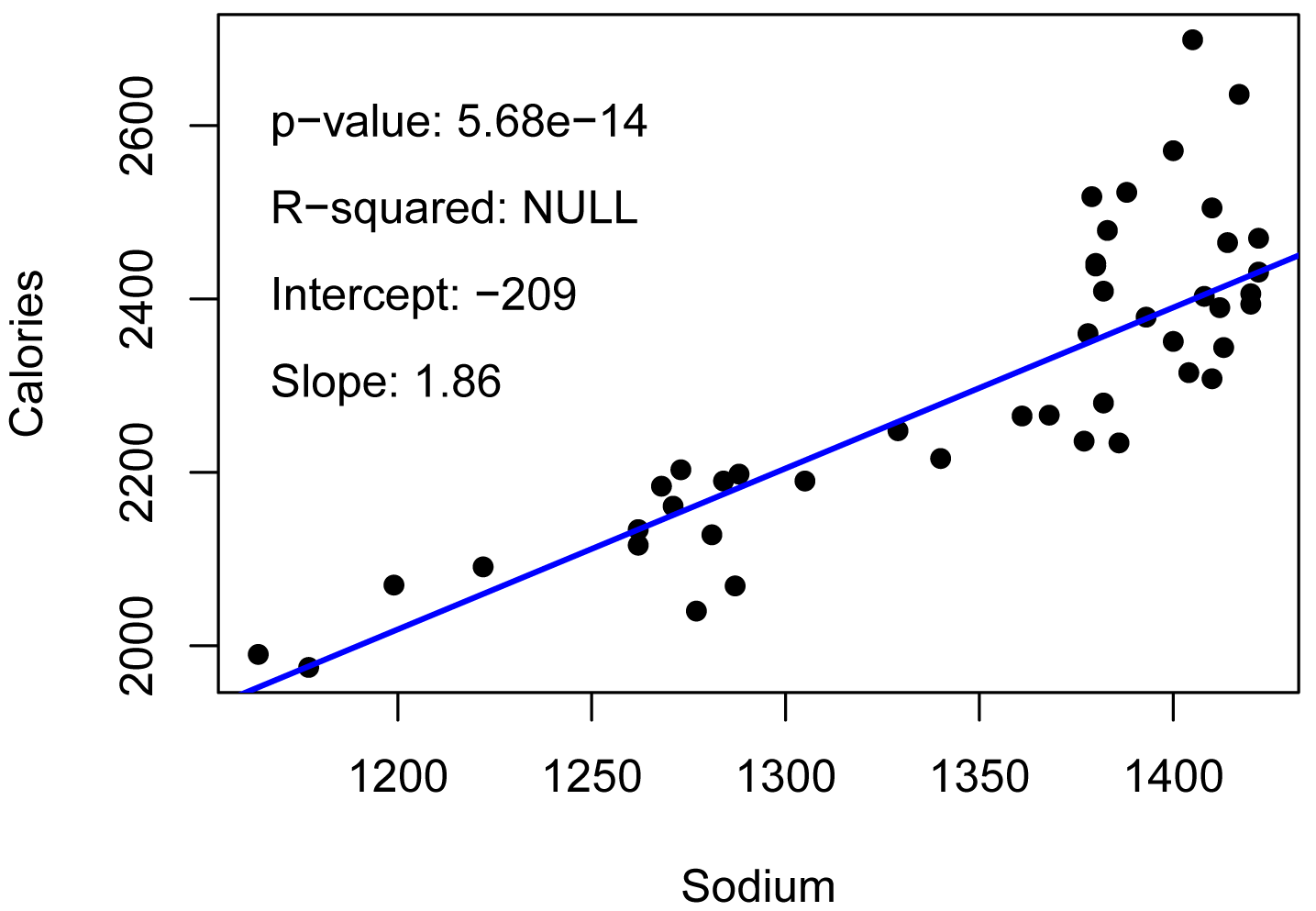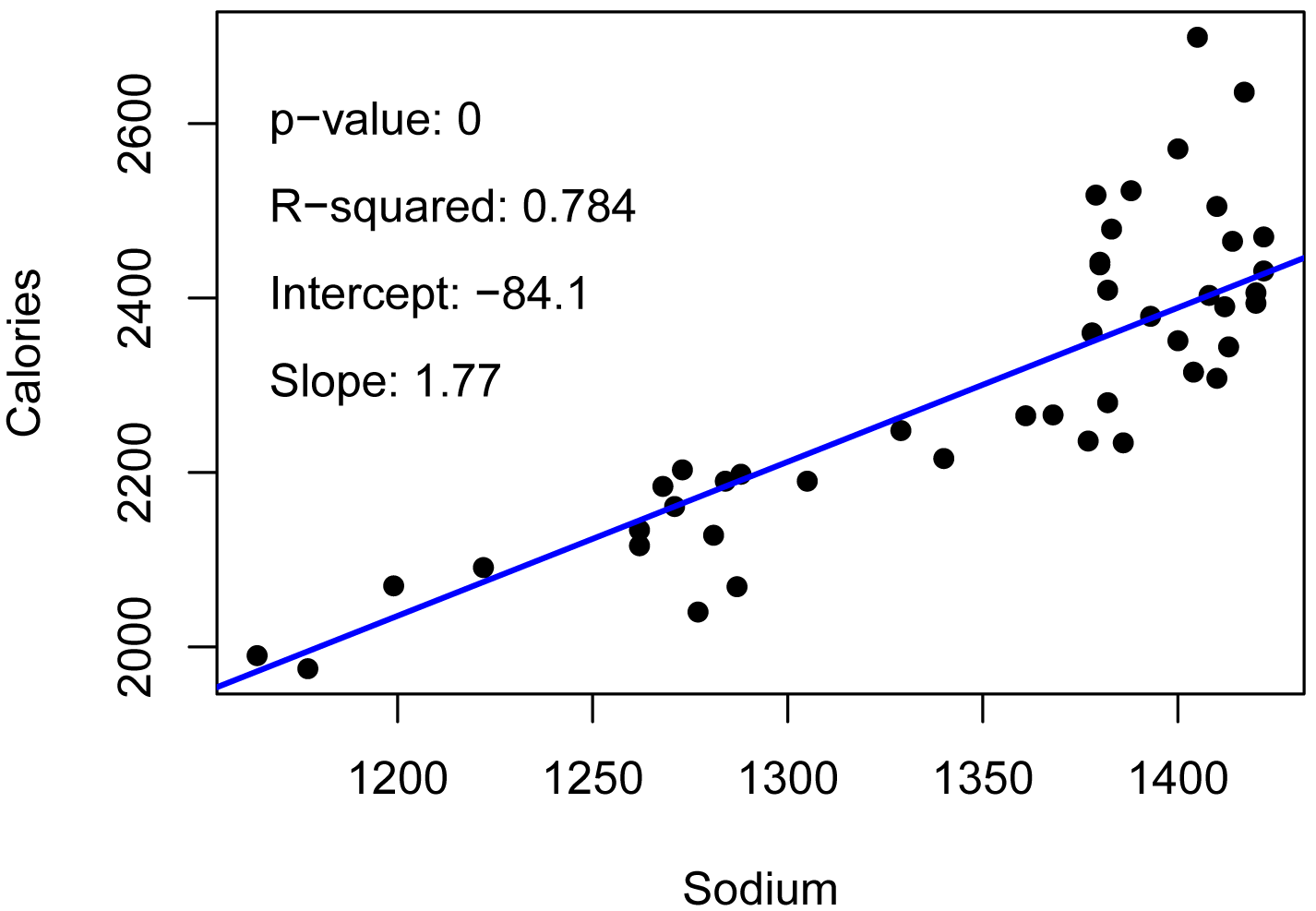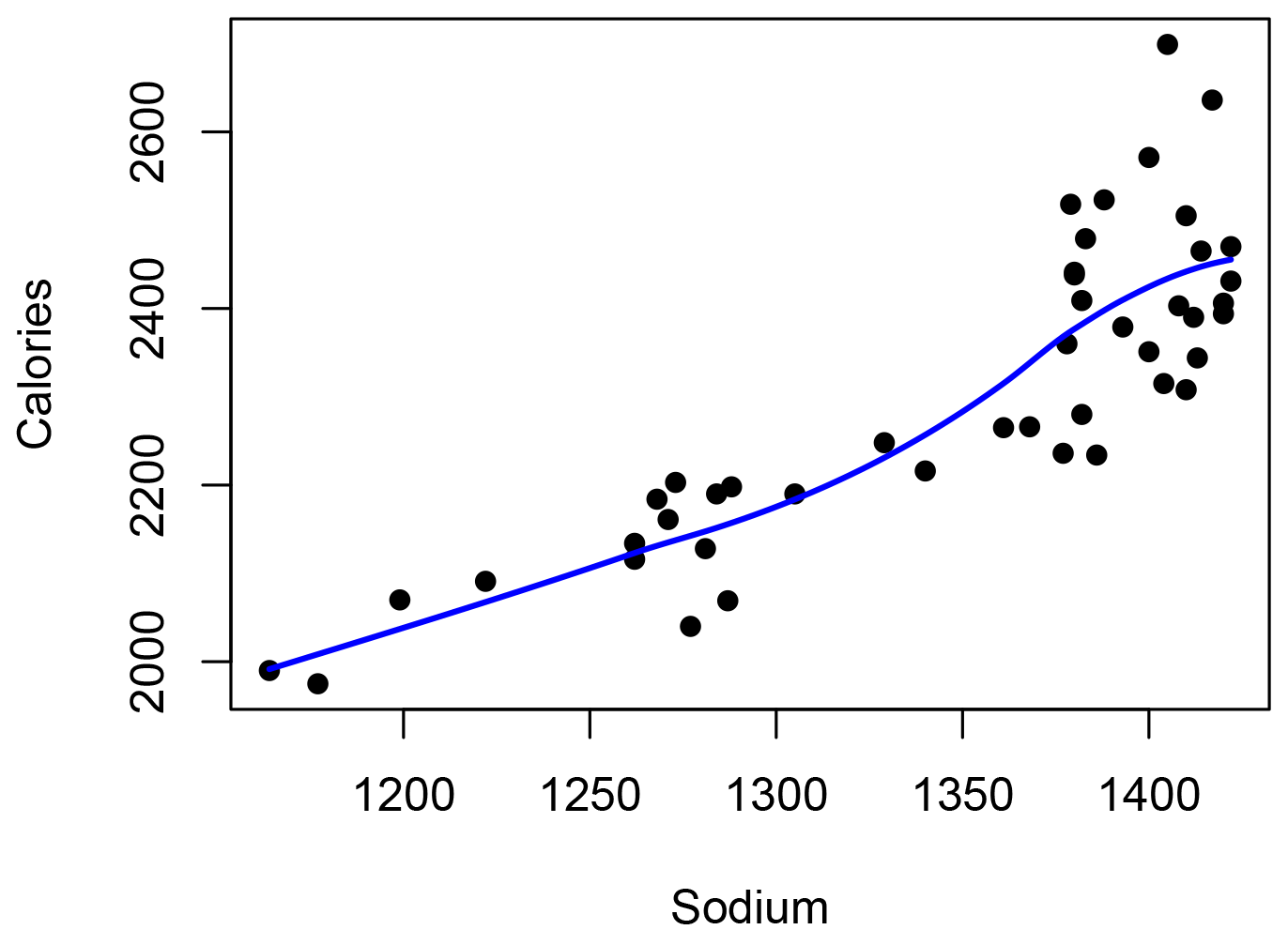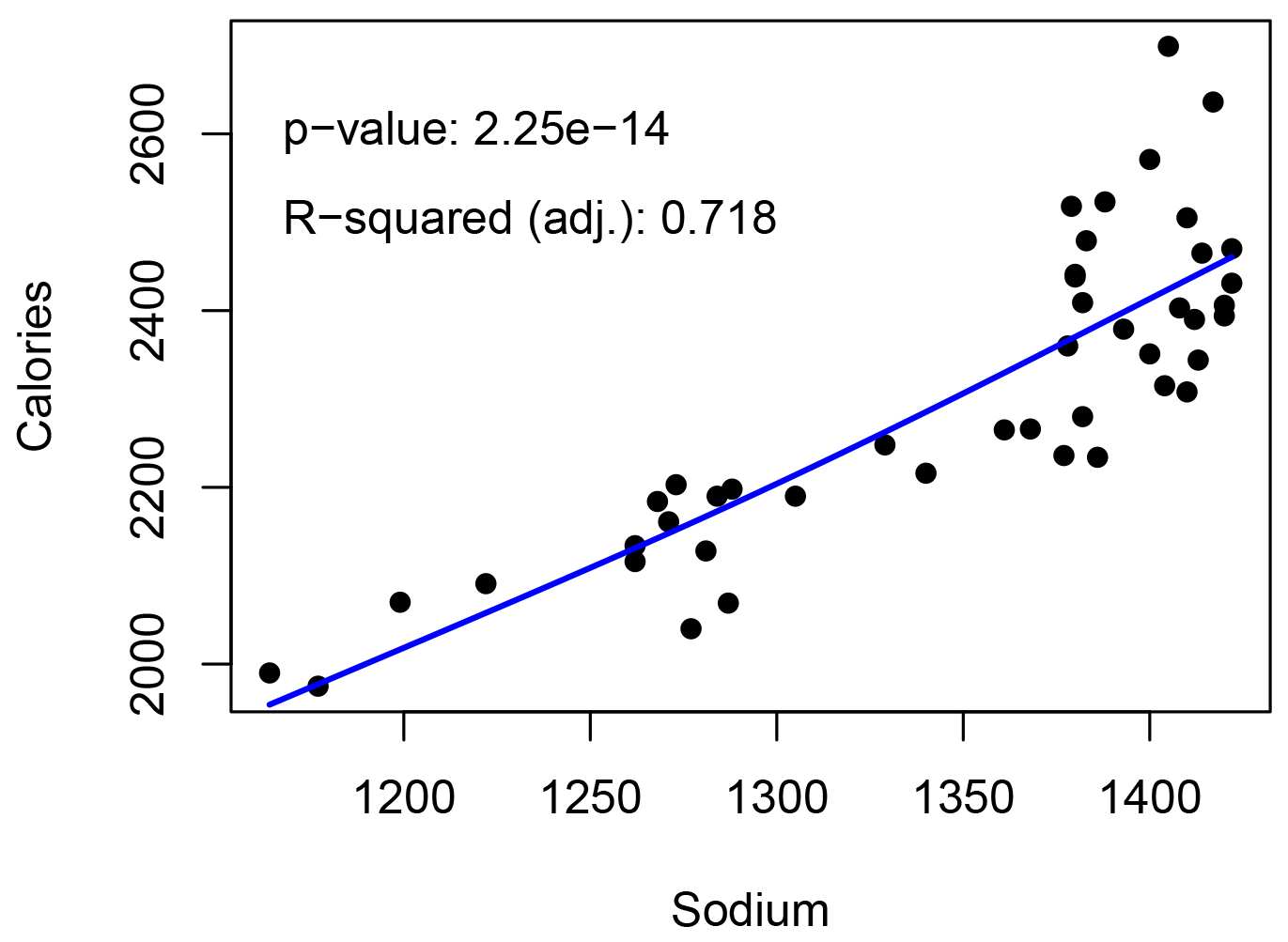## Summary and Analysis of Extension Program Evaluation in R

Salvatore S. Mangiafico

# Nonparametric Regression and Local Regression

There are different techniques that are considered to be forms of nonparametric, semi-parametric, or robust regression.  Kendall–Theil regression fits a linear model between one x variable and one y variable using a completely nonparametric approach.  Rank-based estimation regression is another robust approach.  Quantile regression is a very flexible approach that can find a linear relationship between a dependent variable and one or more independent variables.  Local regression fits a smooth curve to the dependent variable and can accommodate multiple independent variables.  Generalized additive models are a powerful and flexible approach.

### Packages used in this chapter

The packages used in this chapter include:

•  psych

•  mblm

•  quantreg

•  rcompanion

•  mgcv

•  lmtest

•  Rfit

The following commands will install these packages if they are not already installed:

if(!require(psych)){install.packages("psych")}
if(!require(mblm)){install.packages("mblm")}
if(!require(quantreg)){install.packages("quantreg")}
if(!require(rcompanion)){install.packages("rcompanion")}
if(!require(mgcv)){install.packages("mgcv")}
if(!require(lmtest)){install.packages("lmtest")}
if(!require(Rfit)){install.packages("Rfit")}

### Nonparametric correlation

Nonparametric correlation is discussed in the chapter Correlation and Linear Regression.

### Nonparametric regression examples

Data for the examples in this chapter are borrowed from the Correlation and Linear Regression chapter.  In this hypothetical example, students were surveyed for their weight, daily caloric intake, daily sodium intake, and a score on an assessment of knowledge gain

Instructor       Grade   Weight  Calories Sodium  Score
'Brendon Small'     6      43     2069    1287      77
'Brendon Small'     6      41     1990    1164      76
'Brendon Small'     6      40     1975    1177      76
'Brendon Small'     6      44     2116    1262      84
'Brendon Small'     6      45     2161    1271      86
'Brendon Small'     6      44     2091    1222      87
'Brendon Small'     6      48     2236    1377      90
'Brendon Small'     6      47     2198    1288      78
'Brendon Small'     6      46     2190    1284      89
'Jason Penopolis'   7      45     2134    1262      76
'Jason Penopolis'   7      45     2128    1281      80
'Jason Penopolis'   7      46     2190    1305      84
'Jason Penopolis'   7      43     2070    1199      68
'Jason Penopolis'   7      48     2266    1368      85
'Jason Penopolis'   7      47     2216    1340      76
'Jason Penopolis'   7      47     2203    1273      69
'Jason Penopolis'   7      43     2040    1277      86
'Jason Penopolis'   7      48     2248    1329      81
'Melissa Robins'    8      48     2265    1361      67
'Melissa Robins'    8      46     2184    1268      68
'Melissa Robins'    8      53     2441    1380      66
'Melissa Robins'    8      48     2234    1386      65
'Melissa Robins'    8      52     2403    1408      70
'Melissa Robins'    8      53     2438    1380      83
'Melissa Robins'    8      52     2360    1378      74
'Melissa Robins'    8      51     2344    1413      65
'Melissa Robins'    8      51     2351    1400      68
'Paula Small'       9      52     2390    1412      78
'Paula Small'       9      54     2470    1422      62
'Paula Small'       9      49     2280    1382      61
'Paula Small'       9      50     2308    1410      72
'Paula Small'       9      55     2505    1410      80
'Paula Small'       9      52     2409    1382      60
'Paula Small'       9      53     2431    1422      70
'Paula Small'       9      56     2523    1388      79
'Paula Small'       9      50     2315    1404      71
'Coach McGuirk'    10      52     2406    1420      68
'Coach McGuirk'    10      58     2699    1405      65
'Coach McGuirk'    10      57     2571    1400      64
'Coach McGuirk'    10      52     2394    1420      69
'Coach McGuirk'    10      55     2518    1379      70
'Coach McGuirk'    10      52     2379    1393      61
'Coach McGuirk'    10      59     2636    1417      70
'Coach McGuirk'    10      54     2465    1414      59
'Coach McGuirk'    10      54     2479    1383      61
")

###  Order factors by the order in data frame
###  Otherwise, R will alphabetize them

Data\$Instructor = factor(Data\$Instructor,
levels=unique(Data\$Instructor))

###  Check the data frame

library(psych)

str(Data)

summary(Data)

### Kendall–Theil Sen Siegel nonparametric linear regression

Kendall–Theil regression is a completely nonparametric approach to linear regression where there is one independent and one dependent variable.  It is robust to outliers in the dependent variable.  It simply computes all the lines between each pair of points, and uses the median of the slopes of these lines.  This method is sometimes called Theil–Sen.  A modified, and preferred, method is named after Siegel.

The method yields a slope and intercept for the fit line, and a p-value for the slope can be determined as well.  Efron’s pseudo r-squared can be determined from the residual and predicted values.

The mblm function in the mblm package uses the Siegel method by default.  The Theil–Sen procedure can be chosen with the repeated=FALSE option. See library(mblm); ?mblm for more details.

library(mblm)

model.k = mblm(Calories ~ Sodium,
data=Data)

summary(model.k)

Coefficients:
(Intercept) -208.5875  608.4540     230 0.000861 ***
Sodium         1.8562    0.4381    1035 5.68e-14 ***

### Values under Estimate are used to determine the fit line.
### MAD is the median absolute deviation, a robust measure of variability

efronRSquared(model.k)

EfronRSquared
0.71

accuracy(model.k)

\$Fit.criteria
Min.max.accuracy  MAE MedAE   MAPE  MSE RMSE NRMSE.mean NRMSE.median Efron.r.squared CV.prcnt
1            0.972 66.8  48.9 0.0282 8380 91.5     0.0397       0.0397            0.71     3.97

#### Plot with statistics

plot(Calories ~ Sodium,
data = Data,
pch  = 16)

abline(model.k,
col="blue",
lwd=2)

Pvalue    = as.numeric(summary(model.k)\$coefficients[2,4])
Intercept = as.numeric(summary(model.k)\$coefficients[1,1])
Slope     = as.numeric(summary(model.k)\$coefficients[2,1])
R2        = NULL

t1     = paste0("p-value: ", signif(Pvalue, digits=3))
t2     = paste0("R-squared: ", "NULL")
t3     = paste0("Intercept: ", signif(Intercept, digits=3))
t4     = paste0("Slope: ", signif(Slope, digits=3))

text(1160, 2600, labels = t1, pos=4)
text(1160, 2500, labels = t2, pos=4)
text(1160, 2400, labels = t3, pos=4)
text(1160, 2300, labels = t4, pos=4)### Rank-based estimation regression

Rank-based estimation regression uses estimators and inference that are robust to outliers.  It can be used in linear regression situations or in anova-like situations.  The summary function from the Rfit package produces a type of r-squared and a p-value for the model.

library(Rfit)

model.r = rfit(Calories ~ Sodium, data = Data)

summary(model.r)

Coefficients:
Estimate Std. Error t.value   p.value
(Intercept) -213.08411  248.42488 -0.8577    0.3958
Sodium         1.85981    0.18407 10.1036 6.307e-13 ***

Multiple R-squared (Robust): 0.6637139

Reduction in Dispersion Test: 84.86733 p-value: 0

### Quantile regression

While traditional linear regression models the conditional mean of the dependent variable, quantile regression models the conditional median or other quantile. Medians are most common, but for example, if the factors predicting the highest values of the dependent variable are to be investigated, a 95th percentile could be used.  Likewise, models for several quantiles, e.g. 25th , 50th, 75th percentiles, could be investigated simultaneously.

Quantile regression makes no assumptions about the distribution of the underlying data, and is robust to outliers in the dependent variable.  It does assume the dependent variable is continuous.  However, there are functions for other types of dependent variables in the qtools package.  The model assumes that the terms are linearly related. Quantile regression is sometimes considered “semiparametric”.

Quantile regression is very flexible in the number and types of independent variables that can be added to the model.  The example, here, however, confines itself to a simple case with one independent variable and one dependent variable.

This example models the median of dependent variable, which is indicated with the tau = 0.5 option.

A p-value for the model can be found by using the anova function with the fit model and the null model.  A pseudo R-squared value can be found with the nagelkerke function in the rcompanion package.

library(quantreg)

model.q = rq(Calories ~ Sodium,
data = Data,
tau = 0.5)

summary(model.q)

tau:  0.5

Coefficients:
coefficients lower bd   upper bd
(Intercept)  -84.12409   -226.58102  134.91738
Sodium         1.76642      1.59035    1.89615

### Values under Coefficients are used to determine the fit line.
### bd appears to be a confidence interval for the coefficients

model.null = rq(Calories ~ 1,
data = Data,
tau = 0.5)

anova(model.q, model.null)

Quantile Regression Analysis of Deviance Table

Df Resid Df F value    Pr(>F)
1  1       43  187.82 < 2.2e-16 ***

### p-value for model overall

library(rcompanion)

nagelkerke(model.q)

\$Pseudo.R.squared.for.model.vs.null
Pseudo.R.squared
Cox and Snell (ML)                   0.783920
Nagelkerke (Cragg and Uhler)         0.783921

###### Efron’s pseudo r-squared

library(rcompanion)

accuracy(list(model.q))

Efron.r.squared
1            0.707

#### Plot with statistics

plot(Calories ~ Sodium,
data = Data,
pch  = 16)

abline(model,
col="blue",
lwd=2)

library(rcompanion)

Pvalue = anova(model.q, model.null)[][1,4]
Intercept = as.numeric(summary(model.q)\$coefficients[1,1])
Slope     = as.numeric(summary(model.q)\$coefficients[2,1])
R2     = nagelkerke(model.q)[][3,1]

t1     = paste0("p-value: ", signif(Pvalue, digits=3))
t2     = paste0("R-squared: ", signif(R2, digits=3))
t3     = paste0("Intercept: ", signif(coefficients(model), digits=3))
t4     = paste0("Slope: ", signif(coefficients(model), digits=3))

text(1160, 2600, labels = t1, pos=4)
text(1160, 2500, labels = t2, pos=4)
text(1160, 2400, labels = t3, pos=4)
text(1160, 2300, labels = t4, pos=4)### Local regression

There are several techniques for local regression.  The idea is to fit a curve to data by averaging, or otherwise summarizing, data points that are next to one another.  The amount of “wiggliness” of the curve can be adjusted.

Local regression is useful for investigating the behavior of the response variable in more detail than would be possible with a simple linear model.

The function loess in the native stats package can be used for one continuous dependent variable and up to four independent variables.  The process is essentially nonparametric, and is somewhat robust to outliers in the dependent variable.  Usually no p-value is reported.  A pseudo R-squared value can be found with the efronRSquared function in the rcompanion package. Integer variables have to be coerced to numeric variables.

The plot below shows a basically linear response, but also shows an increase in Calories at the upper end of Sodium.

Data\$Sodium = as.numeric(Data\$Sodium)

model.l = loess(Calories ~ Sodium,
data = Data,
span = 0.75,        ### higher numbers for smoother fits
degree=2,           ### use polynomials of order 2
family="gaussian")  ### the default, use least squares to fit

summary(model.l)

Number of Observations: 45
Equivalent Number of Parameters: 4.19
Residual Standard Error: 91.97

efronRSquared(model.l)

EfronRSquared
0.74

accuracy(model.l)

\$Fit.criteria
Min.max.accuracy  MAE MedAE   MAPE  MSE RMSE NRMSE.mean NRMSE.median Efron.r.squared CV.prcnt
1            0.972 67.1  52.7 0.0284 7530 86.8     0.0376       0.0376            0.74     3.76

#### Plot

library(rcompanion)

plotPredy(data  = Data,
x     = Sodium,
y     = Calories,
model = model.l,
xlab  = "Sodium",
ylab  = "Calories")Generalized additive models are very flexible, allowing for a variety of types of independent variables and of dependent variables.  A smoother function is often used to create a “wiggly” model analogous to that used in local regression.  The gam function in the mgcv package uses smooth functions plus a conventional parametric component, and so would probably be classified as a semiparametric approach.  The summary function reports an R-squared value, and p-values for the terms.  The anova function can be used for one model, or to compare two models.

library(mgcv)

model.g = gam(Calories ~ s(Sodium),
data = Data,
family=gaussian())

summary(model.g)

Parametric coefficients:
Estimate Std. Error t value Pr(>|t|)
(Intercept)  2304.87      13.62   169.2   <2e-16 ***

Approximate significance of smooth terms:
edf Ref.df     F  p-value
s(Sodium) 1.347  1.613 66.65 4.09e-15 ***

R-sq.(adj) =  0.718   Deviance explained = 72.6%
GCV = 8811.5  Scale est. = 8352      n = 45

model.null = gam(Calories ~ 1,
data = Data,
family=gaussian())

anova(model.g,
model.null)

Analysis of Deviance Table

Model 1: Calories ~ s(Sodium)
Model 2: Calories ~ 1

Resid. Df Resid. Dev      Df Deviance
1    42.387     356242
2    44.000    1301377 -1.6132  -945135

library(lmtest)

lrtest(model.g,
model.null)

Likelihood ratio test

Model 1: Calories ~ s(Sodium)
Model 2: Calories ~ 1

#Df  LogLik      Df  Chisq Pr(>Chisq)
1 3.3466 -265.83
2 2.0000 -294.98 -1.3466 58.301   2.25e-14 ***

#### Plot with statistics

library(rcompanion)

plotPredy(data  = Data,
x     = Sodium,
y     = Calories,
model = model.g,
xlab  = "Sodium",
ylab  = "Calories")

Pvalue    = 2.25e-14
R2        = 0.718

t1     = paste0("p-value: ", signif(Pvalue, digits=3))
t2     = paste0("R-squared (adj.): ", signif(R2, digits=3))

text(1160, 2600, labels = t1, pos=4)
text(1160, 2500, labels = t2, pos=4)### Note that the fit line is slightly curved.